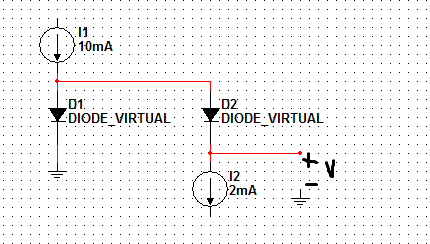# Junction diode problem

asdf12312

## Homework StatementIf the circuit shown, D2= 10 times the junction area of D1. What value of V results? To obtain V=50mV, what current I2 is needed?

## Homework Equations

i(D)=Is(eVD/VT-1)

## The Attempt at a Solution

I know junction area is related to diode current, so D2 has 10 times current as D1. But I still don't really know what to do. I tried KCL equation so 10/11mA -> 0.91mA going through D1. Then current thru D2 = 10*I(D1)=9.09mA this satisfies the KCL equation since 10mA is going on. But I didn't use the equation for diode current I listed so I have a feeling I did this all wrong. Then my other question is how does I2 determine V? It would help if I knew how to find voltage through the diodes but I don't want to assume that it is something like VD=0.7V. the problem didn't say anything about it neither.

## Answers and Replies

Mentor
Choosing I1 and I2 effectively dictates the currents through both diodes. So you can set the currents. Now, the area ratio presumably shows up as differences in the Is for each diode. Is that so? If diode D1 has a saturation current Is, what would be the saturation current for D2?

Can you take your Relevant Equation and write expressions for VD for each diode?

asdf12312
i(D1)=Is(eV/VT-1)
i(D2)=10*Is(eV/VT-1)

because Is increases by same value as area junction A. and then there is the assumptions V=0.7 and VT=25mV (room temperature). But I don't see what else I can do, because part of the current I1 goes through both diodes. I think I have too many unknowns (diode current, Is, V)

Mentor
i(D1)=Is(eV/VT-1)
i(D2)=10*Is(eV/VT-1)

because Is increases by same value as area junction A. and then there is the assumptions V=0.7 and VT=25mV (room temperature). But I don't see what else I can do, because part of the current I1 goes through both diodes. I think I have too many unknowns (diode current, Is, V)

Have faith! Things will cancel.

You've written expressions for the diode currents given their potential drops (V, should be VD. You already have a variable V which stands for the output voltage. Don't confuse things by duplicating meanings for variables!). That's fine, but you'll need to rearrange them so you have expressions for VD in terms of the diode current for each. Note that you're looking for a particular "output" voltage V. So you'll be dealing with the voltages across components. That's why you want to rearrange the expressions so you have the diode voltages in terms of their currents...

You know EXACTLY how much current goes through each diode as soon as you specify I2. KCL applies! What are the two currents iD1 and iD2 in terms of I1 and I2? Can you write then write expressions for the diode voltages in those terms?

asdf12312
Are the values i had in my OP right? I(D1)=I1/11 and I(D2)=10(I1)/11. I could just be overthinking this...if I2=2mA does that mean I(D2) is 2mA and I(D1) is 8mA? Doesn't seem right because I(D2) is supposed to be 10 times I(D1).

Mentor
Are the values i had in my OP right? I(D1)=I1/11 and I(D2)=10(I1)/11. I could just be overthinking this...if I2=2mA does that mean I(D2) is 2mA and I(D1) is 8mA? Doesn't seem right because I(D2) is supposed to be 10 times I(D1).

There's no choice. If I2 is 2mA then 2mA MUST be flowing through D2. That leaves 8mA for D1 by KCL. So those would be the currents IF I2 is chosen to be 2mA. You're looking to find some value of I2 to satisfy a particular V.

So the value of I2 is not known at the outset, you have to find it. In order to do that you need to find the V that results from a choice of I2.

The area relationship for the junctions only tells you what the relative saturation currents are for the diodes. It does not tell you what the actual currents are; those are imposed by the rest of the circuit. The actual currents will produce particular VD's across the diodes according to the diode equation. Those VD's will set the output voltage V. Your mission is to find an I2 that will set the VD's in such a way as to produce that V.

So. Write the expressions for the VD's in terms of the diode currents.